# Tutorial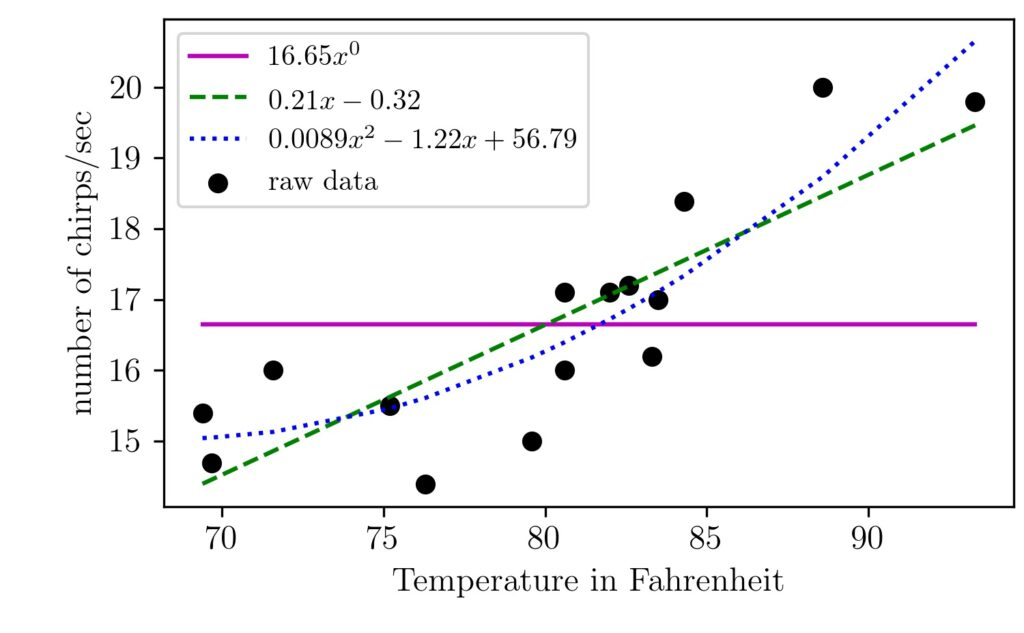## An Intuitive Explanation of Linear Regression

Linear regression is one of the algorithm machine learning enthusiasts start to learn first. In this article, l will walk you through the linear regression intuition. We will implement the basic form of it without using any machine learning packages. The main philosophy of supervised machine learning algorithms is to learn from data. The algorithms …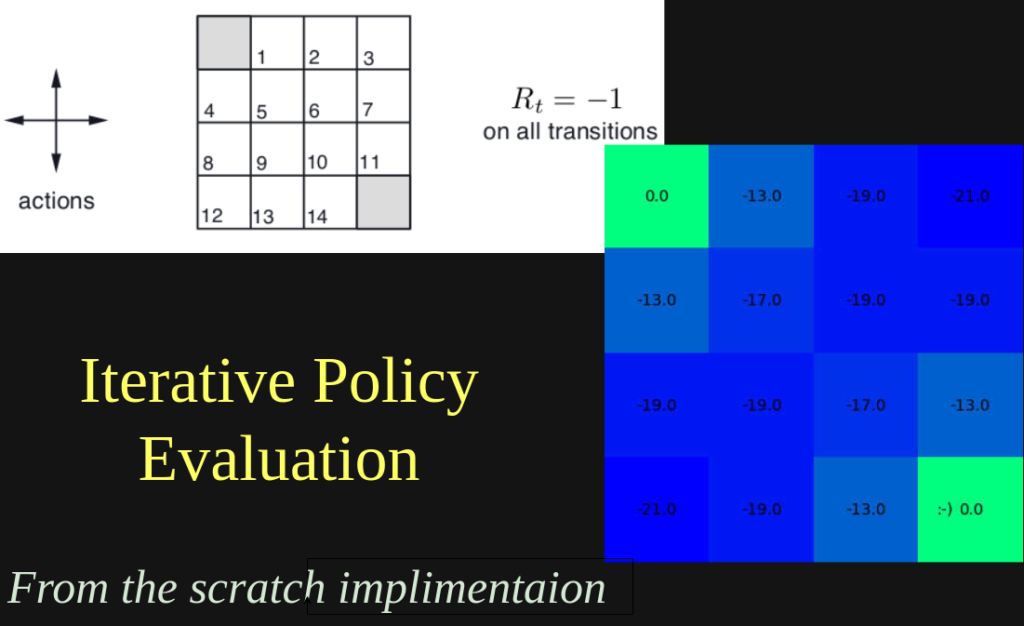## Iterative Policy Evaluation for Estimating Value Function

Introduction In this tutorial, I am going to code the iterative policy evaluation algotithm from the book “Reinforcement Learning: An Introduction by Andrew Barto and Richard S. Sutton”. I am going to take psuedo code, image and examples from this text. The example I am taking for this tutorial is the gird world maze from Chapter …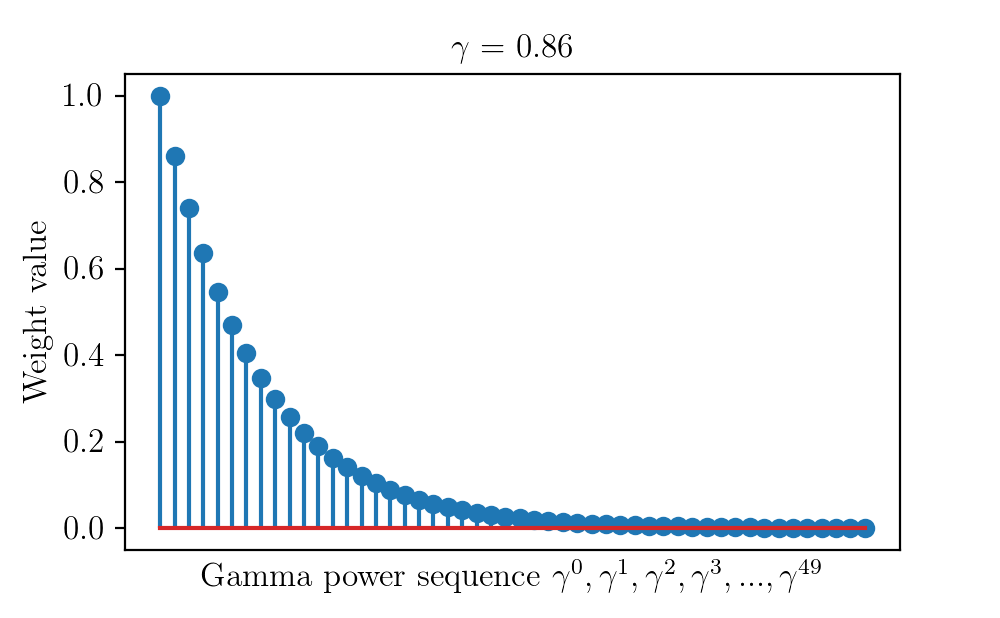## Discount Factor in Reinforcement Learning

This article shows two key visual intuitions behind the usage of a discount factor in reinforcement learning with image, code, and video. Introduction Most of the advances in science and technology happened in the last 100 years. We can see mind-boggling progress in automotive, medicine, communication, energy, etc. . Among these advances, some technologies shake …## LaTeX Tutorial

This tutorial is on the typesetting language called LaTeX. LaTeX is useful when it comes to creating complex documents like thesis, project reports, research articles, etc. We will see the basic use-cases in around 22 examples. LaTeX Installation Instructions Windows In Windows, you need to install two programs called MiKTeX and TeXstudio. Please be careful to install …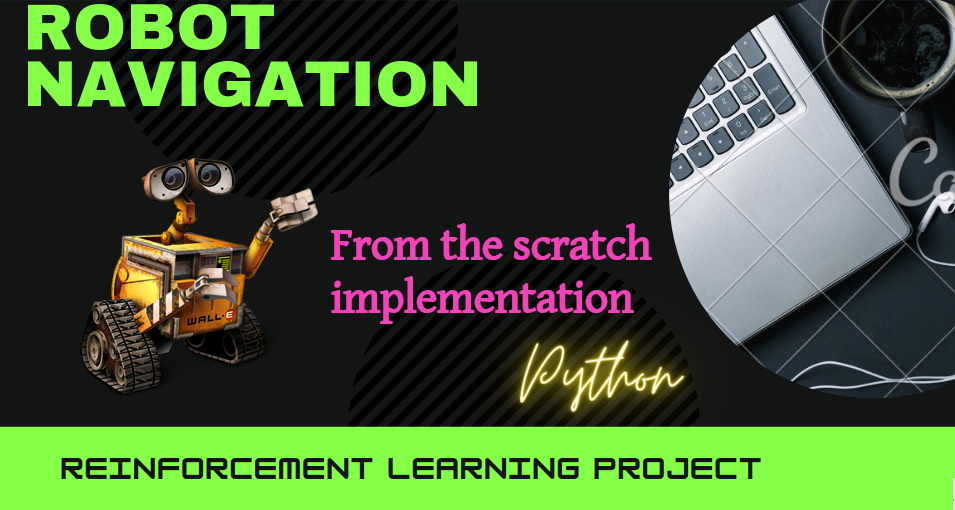## Iterative Policy Improvement

Introduction Iterative Policy Improvement (IPI) is an algorithm in reinforcement learning to find the optimal course of action given the enviroment conditions. This blog post explains how it is done using a simple grid world navigating example. It works by iteratively improving an initial policy using the policy evaluation and policy improvement steps. Here’s how …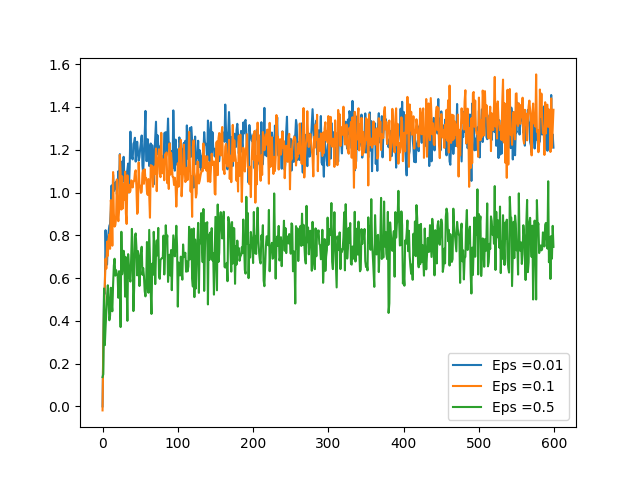## Epsilon Greedy Algorithm in Bandit Problems

Introduction Bandit problems are the simplest possible reinforcement learning scenario. Here the bandit machine can have k arms and pulling each arm leaves the user a reward. One of the arms will be giving higher rewards in the long run and moreover this pattern could be changing over a time period. Think of the scenario …